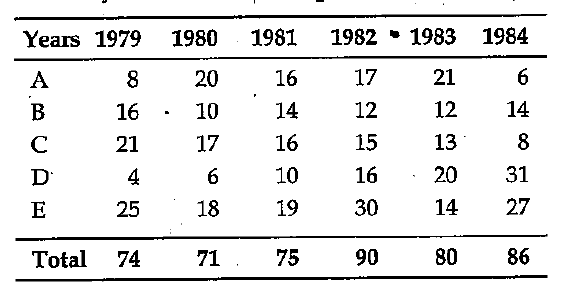# Data Interpretation - Tabular Data

>>>>>>>>Tabular Data

Instructions:

The following table shows the production of cars in a country from 1979 to 1984. Study it carefully and answer the questionsIn which year, the production of all types of cars taken together was approximately equal to the average of the total production during the period?

• A

1979• B

1981• C

1983• D

1984• Option : C
• Explanation : Average of the total production of all types of cars during the period

= (74+71+75+90+80+86)/6

= 476/6 = 79 1/3 = 80 approximately

Instructions:

The following table shows the production of cars in a country from 1979 to 1984. Study it carefully and answer the questions

In which year, the production of all types of cars taken together was approximately equal to the average of the total production during the period?

• A

1980• B

1981• C

1984• D

1983Instructions:

The following table shows the production of cars in a country from 1979 to 1984. Study it carefully and answer the questions

In which year, the production of all types of cars taken together was approximately equal to the average of the total production during the period?

• A

A• B

B• C

C• D

DInstructions:

The following table shows the production of cars in a country from 1979 to 1984. Study it carefully and answer the questions

In which year, the production of all types of cars taken together was approximately equal to the average of the total production during the period?

• A

A• B

B• C

C• D

DInstructions:

The following table shows the production of cars in a country from 1979 to 1984. Study it carefully and answer the questions

In which year, the production of all types of cars taken together was approximately equal to the average of the total production during the period?

• A

15• B

20• C

25• D

30• Option : B
• Explanation : % increase in total production of all types of cars in 1982 to that in 1981 = (15/75)*100 = 20
Related Quiz.
Tabular Data